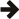Boost C++ Libraries

...one of the most highly regarded and expertly designed C++ library projects in the world.

This is the documentation for an old version of Boost. Click here to view this page for the latest version.Chapter 1. Boost.LocalFunction 1.0.0

Distributed under the Boost Software License, Version 1.0 (see accompanying file LICENSE_1_0.txt or a copy at http://www.boost.org/LICENSE_1_0.txt)

This library allows to program functions locally, within other functions, and directly within the scope where they are needed.

Introduction

Local functions (a.k.a., nested functions) are a form of information hiding and they are useful for dividing procedural tasks into subtasks which are only meaningful locally, avoiding cluttering other parts of the program with functions, variables, etc unrelated to those parts. Therefore, local functions complement other structuring possibilities such as namespaces and classes. Local functions are a feature of many programming languages, notably Pascal and Ada, yet lacking from C++03 (see also [N2511]).

Using C++11 lambda functions, it is possible to implement local functions by naming lambda functions assigning them to local variables. For example (see also add_cxx11_lambda.cpp):

int main(void) {                            // Some local scope.
int sum = 0, factor = 10;               // Variables in scope to bind.

auto add = [factor, &sum](int num) {    // C++11 only.
sum += factor * num;
};

add(1);                                 // Call the lambda.
int nums[] = {2, 3};
std::for_each(nums, nums + 2, add);     // Pass it to an algorithm.

BOOST_TEST(sum == 60);                  // Assert final summation value.
return boost::report_errors();
}

This library allows to program local functions portably between C++03 and C++11 (and with performances comparable to lambda functions on C++11 compilers). For example (see also add.cpp):

int main(void) {                            // Some local scope.
int sum = 0, factor = 10;               // Variables in scope to bind.

void BOOST_LOCAL_FUNCTION(const bind factor, bind& sum, int num) {
sum += factor * num;

add(1);                                 // Call the local function.
int nums[] = {2, 3};
std::for_each(nums, nums + 2, add);     // Pass it to an algorithm.

BOOST_TEST(sum == 60);                  // Assert final summation value.
return boost::report_errors();
}

This library supports the following features for local functions:

• Local functions can capture, or better bind, any of the variables from the enclosing scope (a function together with its captured variables is also called a closure).
• The local function body is programmed using the usual C++ statement syntax (as a consequence, compiler errors and debugging retain their usual meaning and format).
• Local functions can be passed as template parameters so they can be conveniently used with STL algorithms and other templates. 
• However, local functions must be specified within a declarative context (e.g., at a point in the code where local variables can be declared) thus they cannot be specified within expressions. 

See the Alternatives section for a comparison between this library, C++11 lambda functions, Boost.Phoenix, and other C++ techniques that implement features related to local functions.

 This is a strength with respect to C++03 functors implemented using local classes which cannot be passed as template parameters (see [N2657] and the Alternatives section).

 This is a weakness with respect to C++11 lambda functions which can instead be specified also within expressions (see the Alternatives section).

 Last revised: April 13, 2012 at 00:19:19 GMT